# Electronics and Communication Engineering - Exam Questions Papers

16.

If 137 + 276 = 435 how much is 731 + 672?

 A. 534 B. 1403 C. 1623 D. 1513

Explanation:

Given, 137 + 276 = 435

Adding units digits i.e., 7 + 6 = 13, but given as 5, which is 13 - 8 and also 1 is carry forwarded to the tens digit.

i.e., + 1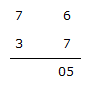Here, 7 + 3 + 1 = 1 i.e., 11 - 8 = 3 and 1 is carry forwarded to hundred digits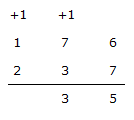Now, the sum of digits in hundred's place is 1 + 1 + 2 = 4

ie.,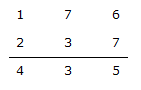Using the same logic, we have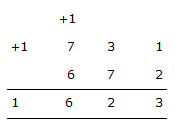Using digits sum 1 + 2 = 3

Tens digits sum = 3 + 7 = 10

i.e., 10 - 2 and + 1 carry forward Hundreds digits sum = 1 + 7 + 6 = 14

i.e., 14 - 8 = 6 and one carry forward.

17.

An astable multivibrator circuit using IC 555 timer is shown below. Assume that the circuit is oscillating steadily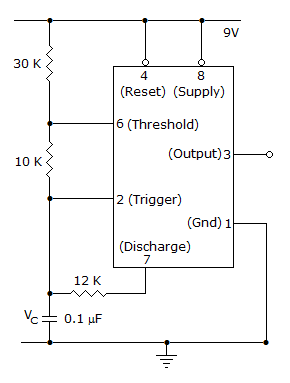The voltage Vc across capacitor varies between

 A. 3 V to 5 V B. 3 V to 6 V C. 3.6 V to 6 V D. 3.6 V to 5 V

Explanation:

Charging and discharging level of capacitor will be the voltage across it.

This is equal to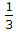Vcc and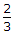Vcc.

Thus 3V to 6V is the voltage VC across the capacitor.

18.

The fourier transform of a double sided exponential function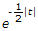is

 A.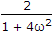B.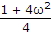C.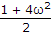D.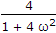Explanation:

Consider general expression

X(jω) =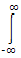ea|t| e- jωt dt

=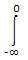eat e-jωt dt +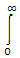e-ate-jωt dt

X(jω) =e(a - jω)t dt +e -(a + jω)t dt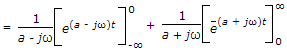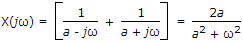Since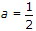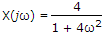.

19.

The number of product terms in the minimized sum of product expression obtained through the following k map is (where "d" denotes don't care states)

 A. 2 B. 3 C. 4 D. 5

Explanation: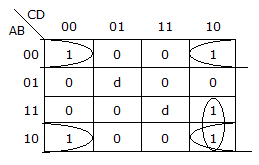Y = A B D + A B D + A C D.

20.

If A =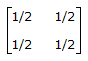then |A50| will be

 A.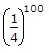(1 - 1002) B.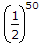(1 - 502) C.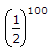(1 - 1002) D.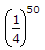(1 - 502)

Explanation:

An = ?

Every n x n matrix satisfy its characteristic equation |A - λI| = 0 λ -> eigen vector

A - λI =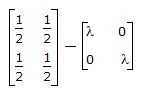|A - λI| =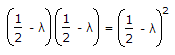= 0

1 =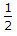,f(A) = An = β0I + β1A

Replace A by 1, I by 1

f(λ) = λn = β0 + β1λ

Differentiate w.r.t. λ nλn - 1 = β1

β1 =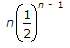β0 = λn - β x λ

β1 =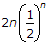β0 =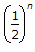-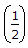=[1 - n]

An =[1 - n]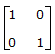+. 2n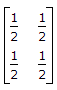An =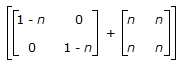=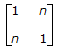A50 =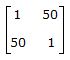|A50| =(1 - 502).

#### Current Affairs 2022

Interview Questions and Answers